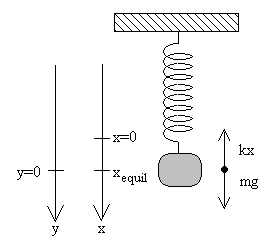## SpringsWhen a mass, m, is hung on the spring it exerts a force due to its weight, W. This force extends the spring a distance, x.     To calculate the spring constant, k, use the formula:

F = -kx

F = force applied (the weight of the mass) in N

k = spring constant in N/m

x = distance the mass moved when the weight was applied in m

The negative sign is there to illustrate a restoring force. In other words, how much force is required to bring the spring back to its original position? The spring constant is NOT negative.

Large spring constants illustrate springs that do not move easily with applied force, such as those in the undercarriage of a car. Light springs, like a Slinky ®, would have a small value for k.

## Horizontal Springs

If a spring is placed on a horizontal tabletop with a mass attached and stretched and then released, it will oscillate from side to side around an equilibrium position…the resting place before it was stretched.In the above diagram, the spring will oscillate around the point where the mass was before elongation. The x (displacement from equilibrium) gives rise to the amplitude of the motion. The spring will oscillate and the spring potential energy will be maximized at the ends of the motion and the kinetic energy will be maximized at the middle (equilibrium) point of the motion. At any point, the total energy of the system will be the sum of spring potential energy and kinetic energy.

Us = K

Us = 1/2 kx2

K = 1/2 mv2

(1) Etot = 1/2 kx2 + 1/2 mv2 at any point in the motion

At the extremes of the motion (the ends), x = A (the amplitude) and K = zero.

(2) Therefore, Etot = 1/2 kA2 (2)

Setting (1) and (2) equal yields,

1/2 kA2 = 1/2kx2 + 1/2 mv2

Cancelling the 1/2, yields

kA2 = kx2 + mv2

Solving for v yields…

v = [(k/m)(A2-x2)]1/2

This equation gives the velocity at any point in the motion. Remember that the velocity is maximized at the equilibrium position and zero at the ends of the motion where spring potential energy is maximized.

To find an expression for the acceleration of the system, consider the two equations:

Fnet = ma and F = kx

Setting them equal yields…

ma = kx OR a = kx/m

So where is a=0? Where x = 0, which is at the equilibrium position and a is maximized where x is the greatest – at the ends where the motion changes direction. The other two variables, k and m are constants.

What does the period of a spring depend upon?

T = 2π(m/k)1/2

It only depends on the mass that is attached and the spring constant, k. The frequency is     the reciprocal of the period: T = 1/f and f = 1/T. T is measured in seconds; f is measured in 1/s or s-1.

(source)